# Java进阶专题(十五) 从电商系统角度研究多线程(下)

## 前言

​ 本章节继上章节继续梳理：线程相关的基础理论和工具、多线程程序下的性能调优和电商场景下多线程的使用。

## 多线程J·U·C

#### 使用

public class ThreadLocalDemo implements Runnable{
public void run(){
for (int i = 0; i < 3; i++) {

);
}
}
public static void main(String[] args) {
}
}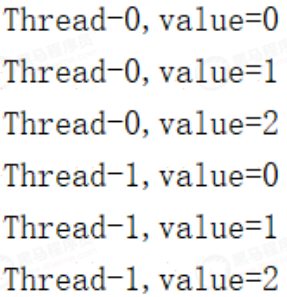#### 使用中遇到的坑

​ 参与过一个项目，电商商铺详情页凌晨调度生成。需要上下传递shopid，为每个商铺重新生成一下。在商铺详情页里因为是按面包屑分片生成，比如商铺信息、热卖商品、最多好评、店主推荐、最新上架等。

#### 源码解析

set方法

public void set(T value) {
//取到当前线程
//从当前线程中拿出Map
if (map != null)
//如果非空，说明之前创建过了
map.set(this, value);
else
//如果没有，那就创建
createMap(t, value);
}


get方法

public T get() {
//获取到当前线程下的Map
if (map != null) {
if (e != null) {
@SuppressWarnings("unchecked")
T result = (T)e.value;
return result;
}
}
//如果map是空的，往往返回一个初始值，这是一个protect方法
return setInitialValue();
}


remove方法

public void remove() {
//很简单，获取到map后，调用remove移除掉
if (m != null)
m.remove(this);
}


### Fork/Join

#### 概念

ForkJoin是由JDK1.7后提供多线并发处理框架。ForkJoinPool由Java大师Doug Lea主持编写，处理逻辑大概分为两步。

1.任务分割：Fork（分岔），先把大的任务分割成足够小的子任务，如果子任务比较大的话还要对子任务进行继续分割。

2.合并结果：join，分割后的子任务被多个线程执行后，再合并结果，得到最终的完整输出。

#### 组成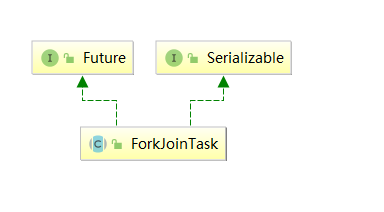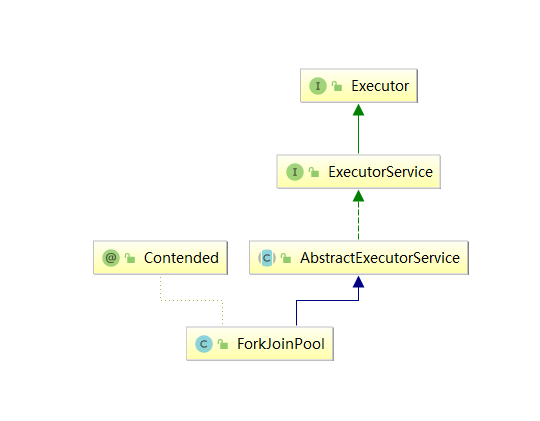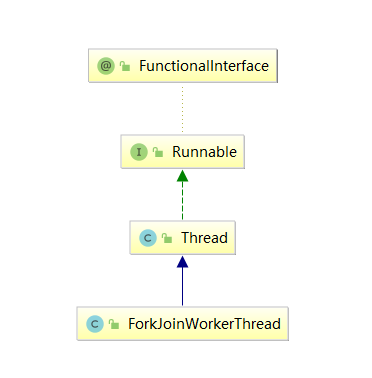• WorkQueue：任务队列，用于保存任务；

#### 基本使用

public class SumTask {
private static final Integer MAX = 100;
// 子任务开始计算的值
private Integer start;
// 子任务结束计算的值
private Integer end;
public SubTask(Integer start , Integer end) {
this.start = start;
this.end = end;
}
@Override
protected Integer compute() {
if(end - start < MAX) {
//小于边界，开始计算
System.out.println("start = " + start + ";end = " + end);
Integer totalValue = 0;
for(int index = this.start ; index <= this.end  ; index++) {
totalValue += index;
}
}else {
//否则，中间劈开继续拆分
}
}
}
public static void main(String[] args) {
ForkJoinPool pool = new ForkJoinPool();
try {
System.out.println("result = " + result);
} catch (InterruptedException | ExecutionException e) {
e.printStackTrace(System.out);
}
}
}


#### 设计思想

• 普通线程池内部有两个重要集合：工作线程集合，和任务队列。
• 队列中的任务被线程执行时，有两种模式，默认是同步模式（asyncMode==false）从队尾取任务（LIFO）
• 窃取：当自己队列中执行完后，工作线程会到其他队列的队首获取任务（FIFO），取到后如果任务再次fork，拆分会被放入当前线程的队列，依次扩张

### Volatile

#### 概念

1. 可见性，是指线程之间的可见性，一个线程修改的状态对另一个线程是可见的
2. 原子性，指的是这个操作是原子不可拆分的，不允许别的线程中间插队操作
3. 有序性指的是你写的代码的顺序要和最终执行的指令保持一致。因为在Java内存模型中，允许编译器和处理器对指令进行重排序，重排序过程不会影响到单线程程序的执行，却会影响到多线程并发执行的正确性。

volatile要解决的就是可见性和有序性问题。

#### 原理

Java内存模型分为主内存和线程工作内存两大类。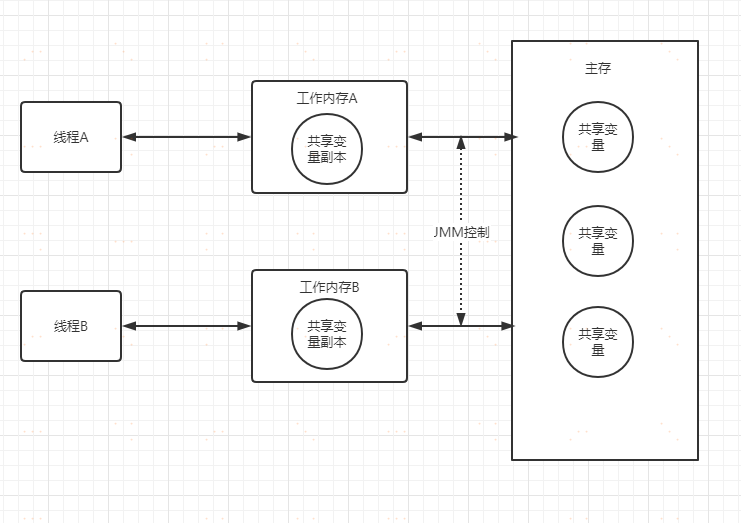​ Java内存模型规定，所有变量都需要存储在主内存中，线程需要时，在自己的工作内存保存变量的副本，线程对变量的所有操作都在工作内存中进行，执行结束后再同步到主内存中去。这里必然会存在时间差，在这个时间差内，该线程对副本的操作，对于其他线程是不见的，从而造成了可见性问题。
​ 但是，当对volatile变量进行写操作的时候，JVM会向处理器发送一条lock前缀的指令，将这个缓存中的变量回写到系统主存中。
​ 同时，在多处理器下，为了保证各个处理器的缓存是一致的，就会实现缓存一致性协议。每个处理器通过嗅探在总线上传播的数据来检查自己缓存的值是不是过期，一旦发现过期就会将当前处理器的缓存行设置成无效状态，强制从主内存读取，这就保障了可见性。
​ 而volatile变量，通过内存屏障可以禁止指令重排。从而实现指令的有序性。

#### 注意

volatile不能保证锁的原子性。

public class BadVolatile {
private static volatile int i=0;
public int get(){
return i;
}
public void inc(){
int j=get();
try {
} catch (InterruptedException e) {
e.printStackTrace();
}
i=j+1;
}
public static void main(String[] args) throws InterruptedException {
for (int i = 0; i < 10; i++) {
public void run() {
counter.inc();
}
}).start();
}
//理论上10才对。可是....
System.out.println(counter.i);
}
}


### ConcurrentHashMap

#### 基本使用

public static void main(String[] args) throws InterruptedException {
//定义ConcurrentHashMap
Map map = new ConcurrentHashMap();
for (int i = 0; i < 10; i++) {
@Override
public void run() {
//多线程下的put可以放心使用
map.put(UUID.randomUUID().toString(), "1");
}
}).start();
}
System.out.println(map);
}


#### 原理

jdk1.7是分段锁,1.8使用的cas+sychronized操作,具体看代码

final V putVal(K key, V value, boolean onlyIfAbsent) {
if (key == null || value == null) throw new NullPointerException();
int binCount = 0;
for (Node<K,V>[] tab = table;;) {//自旋，确保插入成功
Node<K,V> f; int n, i, fh; K fk; V fv;
if (tab == null || (n = tab.length) == 0)
tab = initTable();//表为空的话，初始化表
else if ((f = tabAt(tab, i = (n - 1) & hash)) == null) {
//否则，插入元素，看下面的 casTabAt 方法
//cas 在这里！
if (casTabAt(tab, i, null, new Node<K,V>(hash, key, value)))
break;
}

//...

else {
V oldVal = null;
//其他情况下，加锁保持
//synchronized 在这里！
synchronized (f) {
if (tabAt(tab, i) == f) {
if (fh >= 0) {
binCount = 1;
for (Node<K,V> e = f;; ++binCount) {
K ek;
if (e.hash == hash &&
((ek = e.key) == key ||
(ek != null && key.equals(ek)))) {
oldVal = e.val;
if (!onlyIfAbsent)
e.val = value;
break;
}
Node<K,V> pred = e;
if ((e = e.next) == null) {
pred.next = new Node<K,V>(hash, key, value);
break;
}
}
}
else if (f instanceof TreeBin) {
Node<K,V> p;
binCount = 2;
if ((p = ((TreeBin<K,V>)f).putTreeVal(hash, key,
value)) != null) {
oldVal = p.val;
if (!onlyIfAbsent)
p.val = value;
}
}
else if (f instanceof ReservationNode)
throw new IllegalStateException("Recursive update");
}
}
if (binCount != 0) {
if (binCount >= TREEIFY_THRESHOLD)
treeifyBin(tab, i);
if (oldVal != null)
return oldVal;
break;
}
}
}
return null;
}
//compareAndSetObject，比较并插入，典型CAS操作
static final <K,V> boolean casTabAt(Node<K,V>[] tab, int i,
Node<K,V> c, Node<K,V> v) {
return U.compareAndSetObject(tab, ((long)i << ASHIFT) + ABASE, c, v);
}
//get取值
public V get(Object key) {
Node<K,V>[] tab; Node<K,V> e, p; int n, eh; K ek;
//判断table是不是空的，当前桶上是不是空的
//如果为空，返回null
if ((tab = table) != null && (n = tab.length) > 0 &&
(e = tabAt(tab, (n - 1) & h)) != null) {
//找到对应hash槽的第一个node，如果key相等，返回value
if ((eh = e.hash) == h) {
if ((ek = e.key) == key || (ek != null && key.equals(ek)))
return e.val;
}
//如果正在扩容，不影响，继续顺着node找即可
else if (eh < 0)
return (p = e.find(h, key)) != null ? p.val : null;
//其他情况，逐个便利，比对key，找到后返回value
while ((e = e.next) != null) {
if (e.hash == h &&
((ek = e.key) == key || (ek != null && key.equals(ek))))
return e.val;
}
}
return null;
}


#### 总结：

put过程：
1.根据key的hash值定位到桶位置
2.如果table为空if，先初始化table。
3.如果table当前桶里没有node，cas添加元素。成功则跳出循环，失败则进入下一轮for循环。
4.判断是否有其他线程在扩容，有则帮忙扩容，扩容完成再添加元素。
5.如果桶的位置不为空，遍历该桶的链表或者红黑树，若key已存在，则覆盖，不存在则将key插入到链表或红黑树的尾部。

get过程：
1.根据key的hash值定位到桶位置。
2.map是否初始化，没有初始化则返回null
3.定位的桶是否有头结点，没有返回null
4.是否有其他线程在扩容，有的话调用find方法沿node指针往后查找。扩容与find可以并行，因为node的next指针不会变
5.若没有其他线程在扩容，则遍历桶对应的链表或者红黑树，使用equals方法进行比较。key相同则返回value,不存在则返回null

### 并发容器

1.ConcurrentHashMap

2.CopyOnWriteArrayList

3.CopyOnWriteArraySet

4.ConcurrentSkipListMap

5.ConcurrentSkipListSet

7.BlockingQueue

ArrayBlockingQueue：基于数组实现的可阻塞的FIFO队列
PriorityBlockingQueue：按优先级排序的队列

## 性能调优

### 锁优化

#### Synchronized

public synchronized void test(){
// TODO
}
public void test(){
synchronized (this) {
// TODO
}
}

public static synchronized void test(){
// TODO
}
public static void test(){
synchronized (TestSynchronized.class) {
// TODO
}
}


#### Lock锁优化

public class TotalLock {
//类创建的时间
final long start = System.currentTimeMillis();
//总耗时
AtomicLong totalTime = new AtomicLong(0);
//缓存变量
private Map<String,Long> map = new HashMap(){{put("count",0L);}};
ReentrantLock lock = new ReentrantLock();
//查看map被写入了多少次
lock.lock();
try {
} catch (InterruptedException e) {
e.printStackTrace();
}
long end = System.currentTimeMillis();
//最后操作完成的时间
map.put("time",end);
lock.unlock();
return map;
}
//写入
public Map write(){
lock.lock();
try {
} catch (InterruptedException e) {
e.printStackTrace();
}
//写入计数
map.put("count",map.get("count")+1);
long end = System.currentTimeMillis();
map.put("time",end);
lock.unlock();
return map;
}
public static void main(String[] args) throws InterruptedException {
TotalLock count = new TotalLock();
//读
for (int i = 0; i < 4; i++) {
}).start();
}
//写
for (int i = 0; i < 1; i++) {
count.write();
}).start();
}
System.out.println(count.map);
System.out.println("读写总共耗时："+count.totalTime.get());
}
}


public class ReadAndWrite {
//类创建的时间
final long start = System.currentTimeMillis();
//总耗时
AtomicLong totalTime = new AtomicLong(0);
private Map<String,Long> map = new ConcurrentHashMap(){{put("count",0L);}};
//查看map被写入了多少次
try {
} catch (InterruptedException e) {
e.printStackTrace();
}
long end = System.currentTimeMillis();
//最后操作完成的时间
map.put("time",end);
return map;
}
//写入
public Map write(){
lock.writeLock().lock();
try {
} catch (InterruptedException e) {
e.printStackTrace();
}
//写入计数
map.put("count",map.get("count")+1);
long end = System.currentTimeMillis();
map.put("time",end);
lock.writeLock().unlock();
return map;
}
public static void main(String[] args) throws InterruptedException {
//读
for (int i = 0; i < 4; i++) {
}).start();
}
//写
for (int i = 0; i < 1; i++) {
rw.write();
}).start();
}
System.out.println(rw.map);
System.out.println("读写总共耗时："+rw.totalTime.get());
}
}


#### CAS乐观锁优化

public class NormalSync implements Runnable{
Long start = System.currentTimeMillis();
int i=0;
public synchronized void run() {
int j = i;
//实际业务中可能会有一堆的耗时操作，这里等待100ms模拟
try {
//做一系列操作
} catch (InterruptedException e) {
e.printStackTrace();
}
//业务结束后，增加计数
i = j+1;
" ok,time="+(System.currentTimeMillis() - start));
}
public static void main(String[] args) throws InterruptedException {
NormalSync test = new NormalSync();
System.out.println("last value="+test.i);
}
}
//最终结果是2


public class CasSync implements Runnable{
long start = System.currentTimeMillis();
int i=0;
public void run() {
int j = i;
try {
} catch (InterruptedException e) {
e.printStackTrace();
}
//CAS处理，在这里理解思想，实际中不推荐大家使用！
try {
Field f = Unsafe.class.getDeclaredField("theUnsafe");
f.setAccessible(true);
Unsafe unsafe = (Unsafe) f.get(null);
long offset =
unsafe.objectFieldOffset(CasSync.class.getDeclaredField("i"));
while (!unsafe.compareAndSwapInt(this,offset,j,j+1)){
j = i;
}
} catch (Exception e) {
e.printStackTrace();
}
//实际开发中，要用atomic包，或者while+synchronized自旋
//        synchronized (this){
//            //注意这里！
//            while (j != i){
//                j = i;
//            }
//            i = j+1;
//        }
" ok,time="+(System.currentTimeMillis() - start));
}
public static void main(String[] args) throws InterruptedException {
CasSync test = new CasSync();
System.out.println("last value="+test.i);
}
}
//线程一、二均在100ms+，总耗时200ms，最终结果还是2


### 线程池参数优化

#### 一些经验

1）corePoolSize

2）workQueue

3）maximumPoolSize

4）keepaliveTime

6）handler

#### 并发容器选择

ConcurrentSkipListMap。

posted @ 2020-10-20 17:33  有梦想的老王  阅读(334)  评论(0编辑  收藏